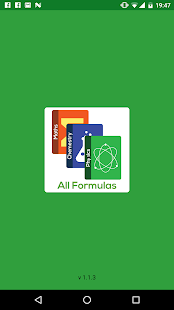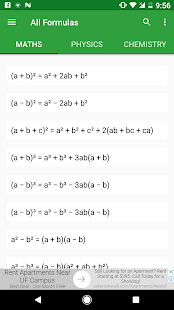# All Formulas – Math, Physics and Chemistry v1.4.7 (Ad-Free) APK [Latest]All Formulas – Math, Physics and Chemistry

A pocket handy formula sheet for maths, physics and chemistry all in the same app, with calculation for necessary formulas.

• You can search any formulas
• You can see the formulas without internet connection.
• You can find all the essential formulas of Mathematics, Physics and Chemistry in one app.
• You can find the details formulas of Math in another app All Math Formulas.
• Periodic table included into Chemistry Formulas section

Screenshots

••